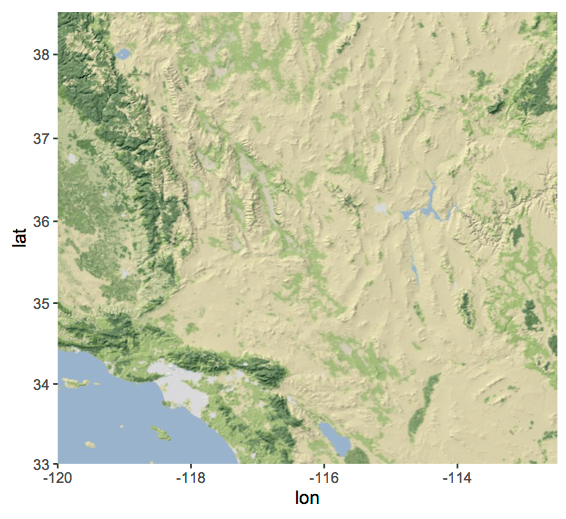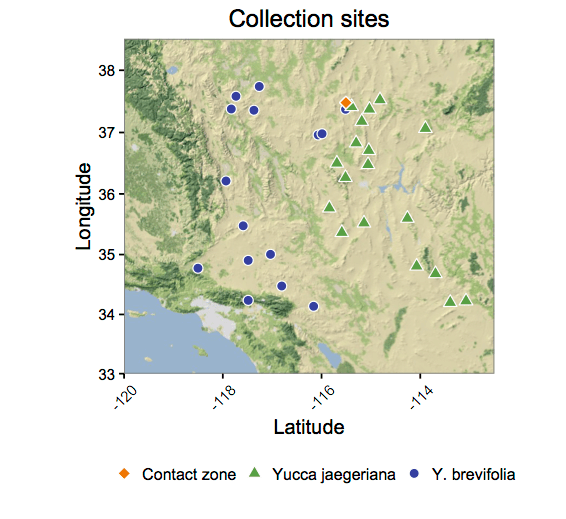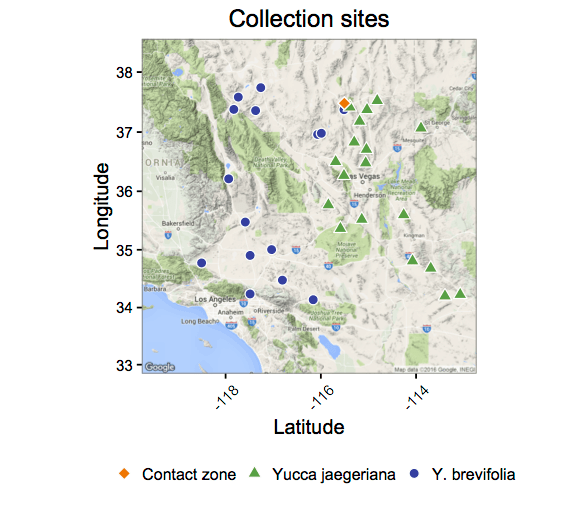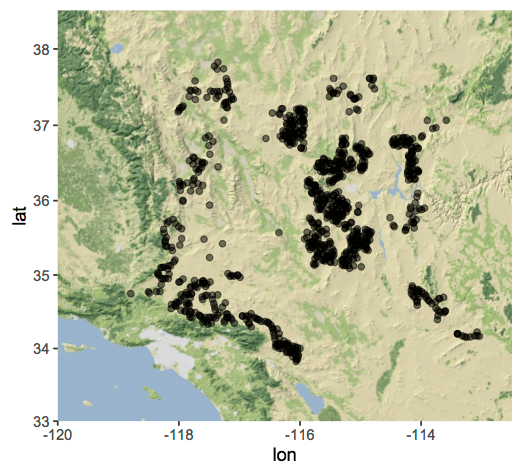# Making Maps in R, volume 2: ggplotsThe open-source statisical programming environment `R` is truly a Swiss Army knife for molecular ecology. With the right code, `R` can processes genetic data and trait measurements, analyze how genetic variants relate to traits, reconstruct phylogenetic trees, and illustrate the results of all that analysis. As molecular ecologists, we’re also interested in placing our analyses of genetic data, trait measurements, and evolutionary history into geographic context. For that, `R` has packages to make maps and estimate models of species distributions.
The map-making capabilities of `R` extend beyond what’s covered in our last post on that subject — in particular, there’s a suite of functions that bring geographic illustrations into the powerful `ggplot2` graphics package, and provide access to publicly available geographic data that can produce professional figures for your next paper or presentation. Here’s a look at a few of those functions.

### Prerequisites

First, run whatever code you run to start up a session of `R` with your preferred settings. Then make sure you’ve installed the `ggplot2` and `ggmap` packages, and a series of others that should look familiar from our prior posts about spatial analysis in `R`:

``````    install.packages("ggplot2")
install.packages("ggmap")
install.packages("maps")
install.packages("mapproj")
install.packages("mapdata")
install.packages("rgeos")
install.packages("maptools")
install.packages("sp")
install.packages("raster")
install.packages("rgdal")
install.packages("dismo")
``````

These installations may take some time, and some of them may install a series of dependencies as well as the requested package. When they’re all done (or if you’ve done the installations before), load the packages into the workspace:

``````    require(ggplot2)
require(ggmap)
require(maps)
require(mapproj)
require(mapdata)
require(rgeos)
require(maptools)
require(sp)
require(raster)
require(rgdal)
require(dismo)
``````

For this demonstration, I’m going to work with the data used in my species distribution modelling demo, a large set of latitude and longitude coordinates for sites where Joshua tree, Yucca brevifolia and Y. jaegeriana, grows — originally published in Godsoe et al. (2008). That dataset is archived in the Dryad repository, and if you have a live internet connection, you can load it directly into `R` from the URL of the raw data file:

``````    bkgd = read.csv("http://datadryad.org/bitstream/handle/10255/dryad.53141/JoTrPresence02202008_dryad.txt", h=T, sep="")
``````

These points are not very evenly dispersed, so it may be useful to “thin” them out a bit by randomly sampling from a grid defined by latitude and longitude, as in the SDMs example:

``````    longrid = seq(-119,-113,0.05) # define the longitude grid
latgrid = seq(33.5,38,0.05) # define the latitude grid
subs = c()
for(i in 1:(length(longrid)-1)){
for(j in 1:(length(latgrid)-1)){
gridsq = subset(bkgd, latitude > latgrid[j] & latitude < latgrid[j+1] & longitude > longrid[i] & longitude < longrid[i+1]) # find points in a given grid square
if(dim(gridsq)>0){
subs = rbind(subs, gridsq[sample(1:dim(gridsq),1 ), ]) # if there's more than one point, draw one at random
}
}
}
dim(subs) # confirm that you have a smaller dataset than you started with
``````

I’m also going to use coordinates for genetic and trait sampling sites from Yoder et al. (2013), another data set archived to Dryad:

``````    locs = read.csv("http://datadryad.org/bitstream/handle/10255/dryad.46089/Yoder_etal_sites.txt", h=T, sep="")
``````

### Plotting sampling sites on a base map

Now we have most of the pieces in place to build maps of Joshua tree’s distribution and the Yoder et al (2013) sampling sites. I’m going to walk through a couple of ways we can do that. First, the `ggmap` package provides a function that downloads basemaps from a number of open-source respositories, including Google Maps — some of those look quite nice right out of the box. To set that up, we first need to know the range of latitudes and longitudes (in decimal degrees) we want to include in the map. In the `locs` dataframe, these are `lat_dec` and `lon_dec`:

``````    range(locs\$lat_dec)
range(locs\$lon_dec)
``````

Those show us latitudes between 34.1368 and 37.7407 degrees north, and longitudes between 113.0736 and 118.5039 degrees west. A range from latitude 33 to 38.5, and from -120 to -112.5 (westing longitudes are denoted as negative, in all these functions) will catch all of those with some elbow room to spare. We use those boundaries to download map images with the `get_map()` function:

``````    base = get_map(location=c(-120,33,-112.5,38.5), zoom=7, maptype="terrain-background")
``````

And then we build a figure using the grammar of `ggplot2`. (If this is unfamiliar, you should really read up on the `ggplot2` package first — this Cookbook for R is a good starting point.)

``````    map1 = ggmap(base)
map1
``````

This plots the “base” map downloaded using `get_map()`.That’s a nice simple terrain map. Let’s add the Yoder et al. (2013) sampling locations, using colors and point shapes to indicate the species of Joshua tree present at each site.

``````    map1 + geom_point(data=locs, aes(x=-lon_dec, y=lat_dec, fill=Type, shape=Type), color="white", cex=2.5) + # plot the points
scale_fill_manual(values = c("darkorange2", "#59A044", "#333FA0"), labels=c("Contact zone", "Yucca jaegeriana", "Y. brevifolia"), name=NULL) +
scale_shape_manual(values = c(23,24,21), labels=c("Contact zone", "Yucca jaegeriana", "Y. brevifolia"), name=NULL) + # define shape/color scales
labs(x="Latitude", y="Longitude", title="Collection sites") + # label the axes
theme_bw() + theme(legend.position="bottom", axis.text = element_text(size = rel(0.75)), legend.key = element_rect(colour = "white"), axis.text.x = element_text(angle=45, vjust=0.5)) # tweak the plot's appearance and legend position
``````

This should give you something like the following figure:You can use other values in the `maptype=` option of `get_map()` to call up different maps as your “base.” Try replacing `terrain-background` with `terrain` in the code above, then re-run it to see what you get.Enter `?get_map` at the `R` command line to call up the helpfile, which lists other options.

### Plotting Joshua tree’s range

So far we’ve only plotted the sampling sites. What about something that gives us a sense of where those sites fall in Joshua tree’s actual distribution? For that, we could just plot the sub-sampled “background” points you should already have in memory as `subs`:

``````    map1 + geom_point(data=subs, aes(x=longitude, y=latitude), alpha=0.5)
``````Note that latitude and longitude are in columns with different names in `subs`, and that there’s no need to take the opposite of the westing longitudes — they’re already negative. (This is the fun of working with multiple data sources!) We can add the sampling sites on top of the semi-transparent background points like this:

``````    map1 + geom_point(data=subs, aes(x=longitude, y=latitude), alpha=0.5) +
geom_point(data=locs, aes(x=-lon_dec, y=lat_dec, fill=Type, shape=Type), color="white", cex=2.5) +
scale_fill_manual(values = c("darkorange2", "#59A044", "#333FA0"), labels=c("Contact zone", "Yucca jaegeriana", "Y. brevifolia"), name=NULL) +
scale_shape_manual(values = c(23,24,21), labels=c("Contact zone", "Yucca jaegeriana", "Y. brevifolia"), name=NULL) +
labs(x="Latitude", y="Longitude", title="Collection sites") +
theme_bw() + theme(legend.position="bottom", axis.text = element_text(size = rel(0.75)), legend.key = element_rect(colour = "white"), axis.text.x = element_text(angle=45, vjust=0.5))
``````That’s functional, if not very attractive. What might be nicer would be to generate polygons that surround the area covered by the presence points. We can do this using a function from the `dismo` package, `circles()`, which draws circles of a given radius, centered on a set of points — and can merge overlapping circles into larger polygons.
First, use `circles()` to generate the polygons, as a complex spatial data object. I’m using coordinates from the full `bkgd` dataframe, generating circles with a radius of 5,000 meters (the option `d=5000`), and “dissolving” overlapping circles into larger merged polygons (`dissolve=T`).

``````    x = circles(bkgd[,c("longitude","latitude")], d=5000, lonlat=T, dissolve=T)
``````

Then, I convert the spatial data object `x` into a dataframe that `ggplots` can interpret:

``````    p = fortify(polygons(x))
``````

And now we can use that dataframe to plot the polygons on our base map using `geom_poly()`, as dark-olive shaded areas.

``````    map1 + geom_polygon(data=p, aes(x=long, y=lat, group=group), alpha=0.75, fill="darkolivegreen", color=NA)
``````Finally, we can put that together with the previous code to plot the Yoder et al. (2013) sampling sites:

``````    map1 + geom_polygon(data=p, aes(x=long, y=lat, group=group), alpha=0.75, fill="darkolivegreen", color=NA) +
geom_point(data=locs, aes(x=-lon_dec, y=lat_dec, fill=Type, shape=Type), color="white", cex=2.5) +
scale_fill_manual(values = c("darkorange2", "#59A044", "#333FA0"), labels=c("Contact zone", "Yucca jaegeriana", "Y. brevifolia"), name=NULL) +
scale_shape_manual(values = c(23,24,21), labels=c("Contact zone", "Yucca jaegeriana", "Y. brevifolia"), name=NULL) +
labs(x="Latitude", y="Longitude", title="Collection sites") +
theme_bw() + theme(legend.position="bottom", axis.text = element_text(size = rel(0.75)), legend.key = element_rect(colour = "white"), axis.text.x = element_text(angle=45, vjust=0.5))
``````## About Jeremy Yoder

Jeremy B. Yoder is an Assistant Professor of Biology at California State University Northridge, studying the evolution and coevolution of interacting species, especially mutualists. He is a collaborator with the Joshua Tree Genome Project and the Queer in STEM study of LGBTQ experiences in scientific careers. He has written for the website of Scientific American, the LA Review of Books, the Chronicle of Higher Education, The Awl, and Slate.
This entry was posted in howto, R, software and tagged , . Bookmark the permalink.